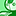Announcements
Applications are open for the 2024 Qlik Luminary Program. Apply by December 15 here.
cancel
Showing results for
Did you mean:Creator

## Divide one expression from another?!

I have a straight table with multuple columns which have expression associated with them e.g.

Column 2

Sum([Working Days]) - sum({<Task={'Personal Holiday', 'Sickness', 'Contractor Leave'}>} Days)

Column 3

=sum({<[Working Day]={'Yes'}>} Days)

In column 1 I wish to show the results of Column 3 divided by Column 2. I have performed a workaround by including the following:

=Column(3) / Column (2)

But I wish to utilise the calculation else where in other charts so this wont work. is there a way of doing this with sum of expression within a wide expression?

The result would be a %

I tried the following but am returning the incorrect results:

=sum({<[Working Day]={'Yes'}>} Days)/Sum([Working Days]) / sum({<Task={'Personal Holiday', 'Sickness', 'Contractor Leave'}>} Days)

Thanks

Martin

Labels (2)

• ### Qlik Sense

1 Solution

Accepted SolutionsPartner - Champion

maybe this:
=sum( {< [Working Day] = {'Yes'} >} Days )
/
(
Sum( [Working Days] ) - sum( {< Task = {'Personal Holiday', 'Sickness', 'Contractor Leave'} >} Days )
)
-1

the result will be a percentage %.

2 RepliesPartner - Champion

maybe this:
=sum( {< [Working Day] = {'Yes'} >} Days )
/
(
Sum( [Working Days] ) - sum( {< Task = {'Personal Holiday', 'Sickness', 'Contractor Leave'} >} Days )
)
-1

the result will be a percentage %.Creator
Author

many thanks this worked perfectly. only amendment i made was the removal of the -1 as the column i inserted within was a measure.

thanks

MartinTags
Community Browser